0 Cart
You have items in your cart
You have 1 item in your cart
Total

# 8 fun multiplication games for your math centers

by Colleen Uscianowski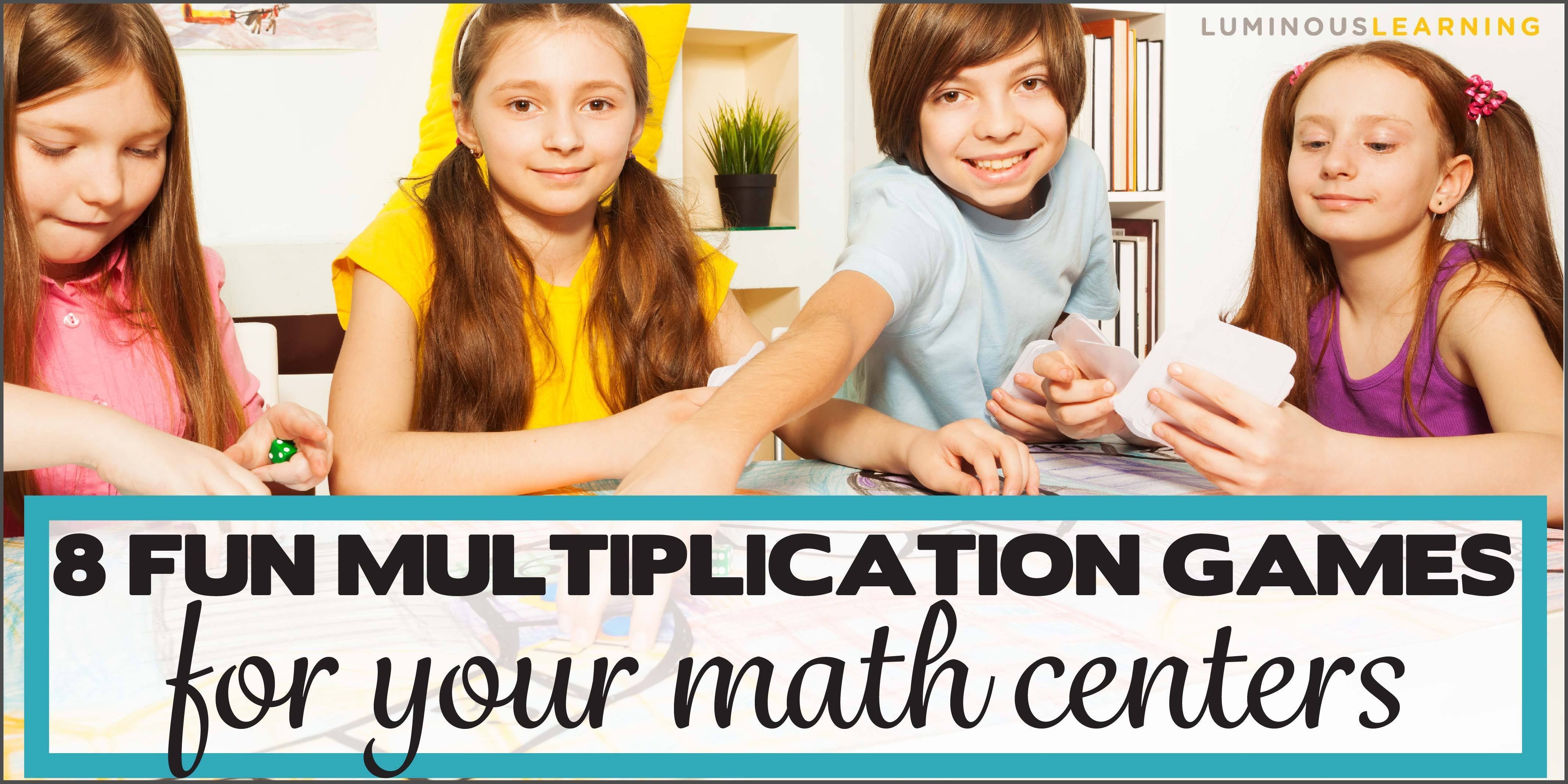I LOVE playing games (not gonna lie, I've been known to get a little too serious about a game or two of Monopoly).

But it's not just me. My students LOVED playing games. I've never seen such an engaged group as when we played math games during Funday Friday.

Do you want to know the best thing about math games for the classroom?

Math games are not only fun, but they're an incredibly good way to practice math skills! They turn boring ol' math practice into a fun, motivational exercise. In fact, games are so much fun that students don't even know that they're learning!

Sshhh..just don't tell my students.

I want to share 8 fun multiplication games that you can use in your math centers. One reason I love these multiplication games is because they don't require you to buy anything special. You can use materials you already have in your classroom or at home.

So dig out the dice, playing cards, math counters, and let's play some math games!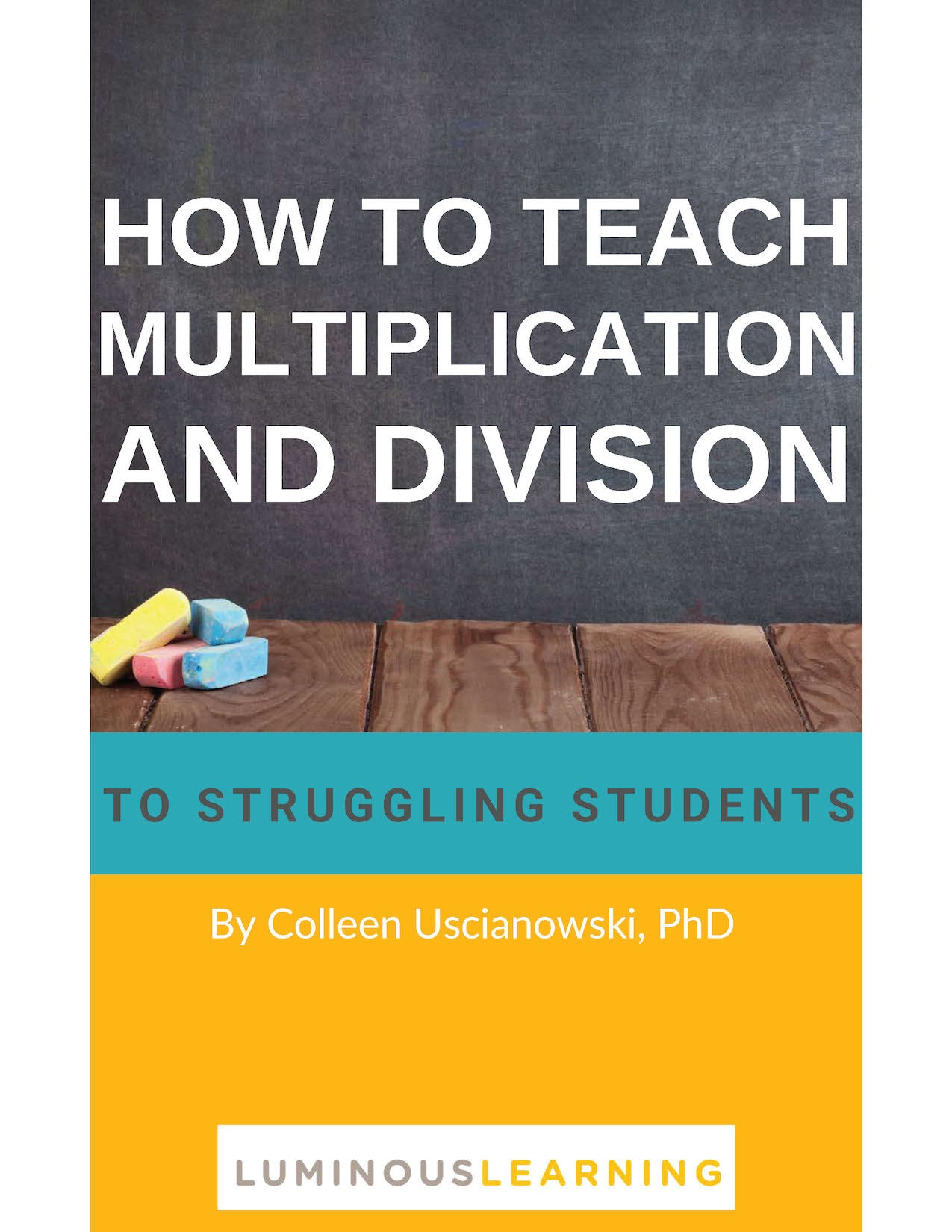# How to Teach Multiplication and Division ebook

Get your FREE 20+ page guide to teaching multiplication in the special education classroom + printable activities!

## Math games for each phase of math learning

In this post, we talked about different phases of multiplicative thinking that students progress through on the way towards multiplication mastery.

In the concrete phase, students are learning the conceptual meaning of multiplication. In this phase, they benefit from multiplication practice with hands-on manipulatives, such as counters and unifix cubes.

In the representational phase, students understand what it means to multiply and now they're learning to apply more strategic ways of solving multiplication problems, such as using drawing arrays and skip counting to find the answer. In this phase, they benefit from multiplication practice that involves drawing pictures or diagrams.

In the abstract phase, students are solving multiplication problems without the use of manipulatives or drawings. They are practicing different efficient strategies to solve problems, such as relying on known facts. In this phase, students benefit from practice recalling math facts from memory while playing fun games, such as multiplication war.

The multiplication games I describe below can be categorized into one of these 3 phases of multiplication development. Some games should be used when students are first learning to multiply and needs hands-on practice, while other games are better for students who have been learning multiplication for awhile but need more practice recalling the facts from memory.

One quick note: I find that some of my special education students, especially those are math anxious or math averse, don't enjoy playing games where there's a winner and a loser. They can feel especially defeated if they end up losing a game, which defeats the purpose of getting them more excited about math! In this case, I'll turn the following games into activities and take out the winning and losing aspect.

## Multiplication games for the concrete phase

### Multiplication arrays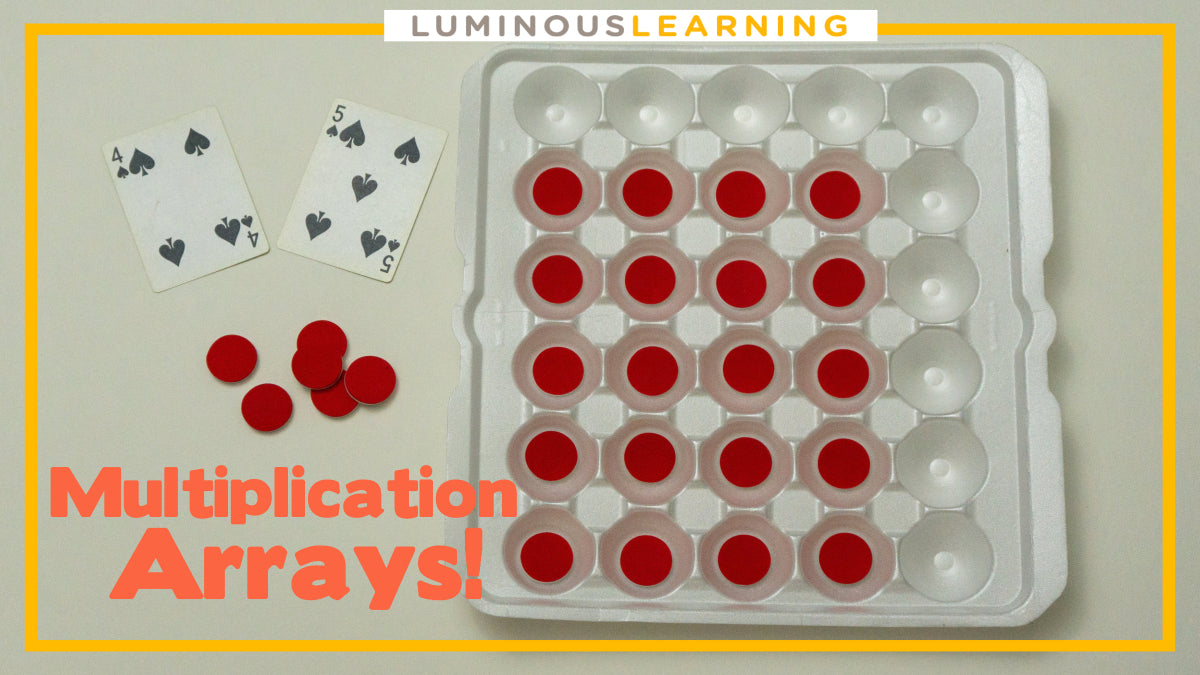Description:

This is a math game with playing cards and counters. Students create arrays that represent multiplication equations. Play with 2 - 4 students in each group.

Materials:

• math counters
• deck of playing cards (depending on the size of the carton, you should remove some of the playing cards. For example, the carton above only has 5 columns and 6 rows so I would take out any cards greater than 6).
• a large carton (I can usually find good sized cartons in bulk warehouse stores, such as Costco. Look for large containers that store eggs or apples.)

Directions:

• Each student takes 2 cards from the deck of playing cards. The first card they turn over represents the number of columns. The second card they turn over represents the number of rows.
• Students use the counters to create an array. In the example above, the student created an array using red counters with 4 rows and 5 columns.
• The student with the largest product wins that round and collects all the cards.
• Next, students should remove all counters from their array and start from the top. Each student should turn over another 2 cards and build a new array.
• The student with the most cards at the end of the game wins the game!
• Pro tip: Instead of using counters, I sometimes use pennies when playing this game. I find that some of my students with math disabilities grasp math more easily when it's put in terms of money. Instead of counting all the counters in the example above, students can count all the pennies to calculate that 5 rows of 4 pennies equals 20 pennies in all.

### Multiplication groups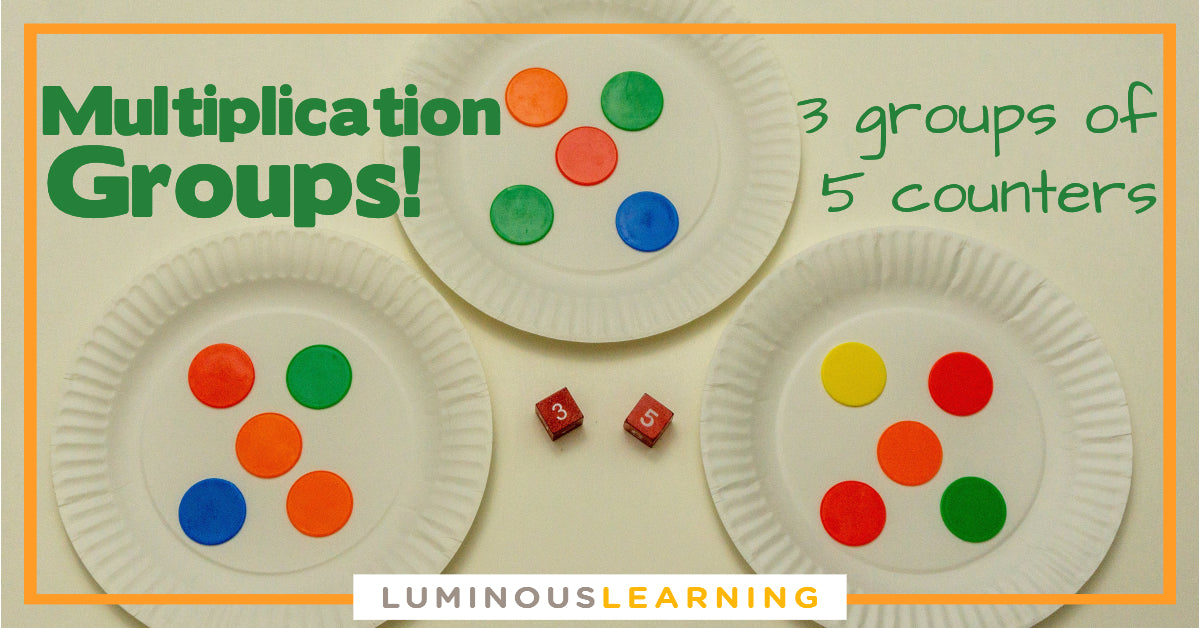Description:

This multiplication game uses counters, dice, and paper plates. Students roll the dice and create groups that represent multiplication equations. Play with 2 students per group.

Materials:

• math counters
• paper plates
• 4 dice
• paper and pencil to keep score

Directions:

• Each student rolls 2 dice. The first number they roll represents the number of groups and the second number represents the number of counters in each group. In the example above, the student rolled a 3 and a 5, which represents 3 groups of 5 counters.
• Students take as many paper plates as they need to make groups. If I roll a 3 first, I will take 3 plates to make 3 groups.
• Students take as many counters as they need to fill up the plates. If I roll a 5, I will place 5 counters on each plate.
• Students count to find the total number of counters. In the example above, the student make 3 groups of 5 counters, which is 15 counters altogether.
• The student with the greatest number of counters wins this round and gets a point. Students should keep track of their points on a separate sheet of paper.
• Put back the counters and begin again from step #1. Students continue to roll the dice and make groups with counters. You can continue this game for as long as you want, although I find that about 15 minutes is a good amount of time. The student with the most number of points at the end of the game is the winner!

## Multiplication games for the representational phase

### Multiplication Stacks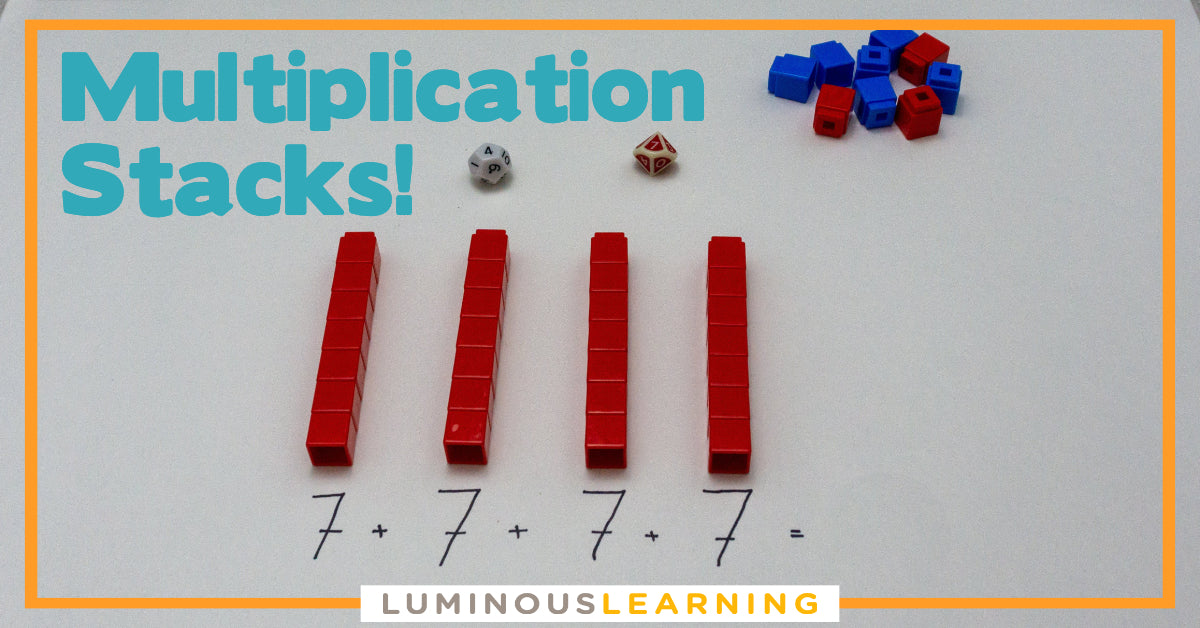Description:

In this math game, students roll the dice and create stacks of unifix cubes. They use repeated addition or skip counting to solve the multiplication problem and find the total number of cubes. Play with 2 - 4 students in each group.

Materials:

• unifix cubes or another type of linking cube
• 2 dice for each student
• dry erase board
• paper and pencil to keep score

Directions:

• Each student rolls their pair of dice. The first number they roll tells them how many stacks to make. The second number they rolls tells them how big to make each stack.
• Students stack their unifix or linking cubes to make their stacks. In the example above, the student rolled a 4 and a 7 so they made 4 stacks of 7 unifix cubes each.
• Students skip count or use repeated addition to find the total number of unifix cubes they stacked. They should show their work on the dry erase board.
• The student with the largest product wins this round and gets a point. Students should keep track of their points on a separate sheet of paper.
• Begin again from step #1. Students continue to roll the dice and make stacks with linking cubes. You can continue this game for as long as you want, although I find that about 15 minutes is a good amount of time. The student with the most number of points at the end of the game is the winner!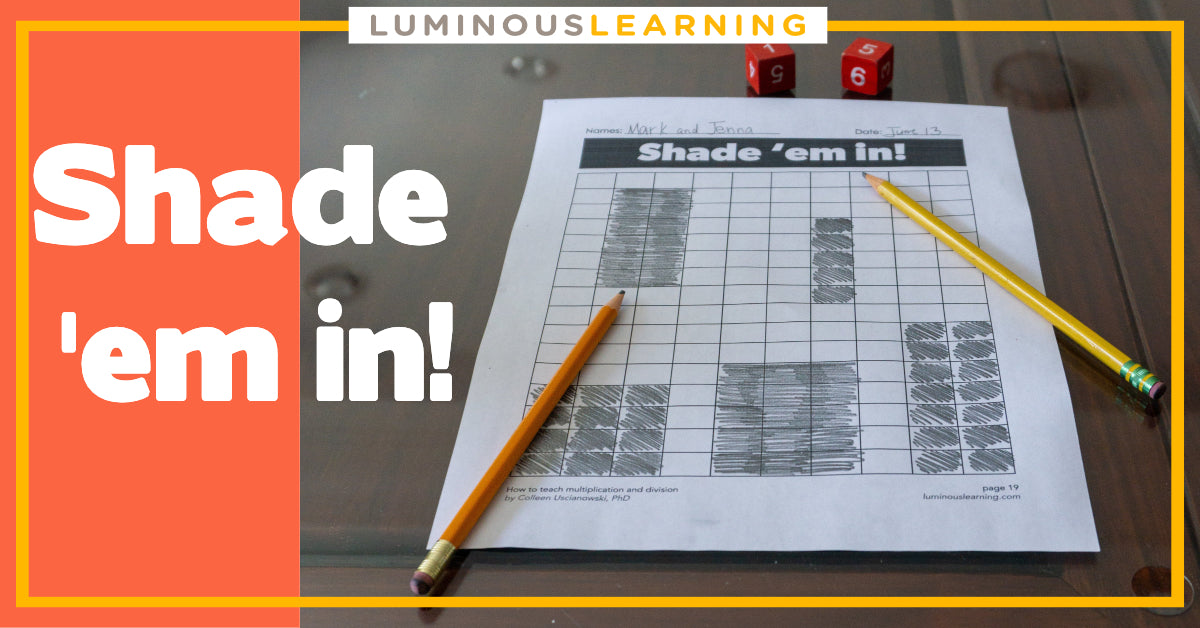Description:

Students shade in multiplication arrays on graph paper in this math game. They roll the dice to find out how many rows and columns to make their array. Play with 2 students in each group.

Materials:

• graph paper (you can also use my printable graph paper which I give for free as part of my How to Teach Multiplication and Division Ebook. Download my ebook below for a free copy of Stack 'em up!)
• regular pencil or colored pencil
• Pair of dice

Directions:

• Students take turns. The first student rolls the two dice, which represent the rows and columns in an array.
• The first student shades in the correct number of rows and columns anywhere on the graph paper. In the example above, the student just rolled a 1 and a 5. They can shade in 1 row and 5 columns or 5 rows and 1 column.
• Now it's the second student's turn. The second student rolls the dice and shades in the array represented by the dice.
• The students continue taking turns rolling the dice and shading in arrays. The first student who runs out of room and cannot find a place to shade in their array loses. The other student is the winner!

## Multiplication games for the concrete phase

### Multiplication war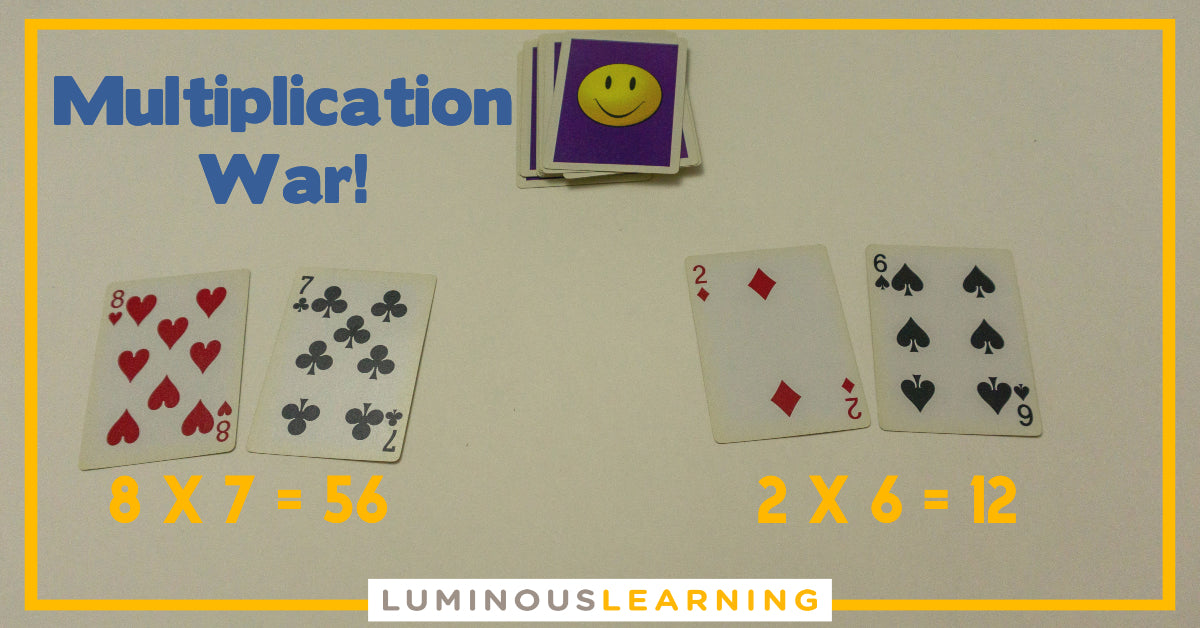Description:

I declare war! A spin on the classic game, students turn over two cards each and multiply the numbers on the cards. Play this math card game with 2 - 4 students per group (although if you play with more than 2 students, you should combine 2 decks of playing cards).

Materials:

• deck of playing cards (you can make the A a 1 and remove the J, Q, and K. Alternatively, if your students are ready to multiply larger numbers, you can make the J a 10, the Q an 11, and the K a 12.)

Directions:

• Shuffle the cards and turn them upside in a pile.
• Students take turns taking two cards from the top of the pile.
• Students turn over their cards. The number on each card represents the factors they need to multiply. Students multiply their factors and say the product aloud. For example, if I turned over an 8 and a 7, I would say: 8 x 7 equals 56.
• The student with the largest product wins this round and collects everyone's cards.
• Go back to step #1. Students continue multiplying two playing cards until there are no cards left. The student who collected the most cards by the end of the game is the winner!

### Multiplication dominoes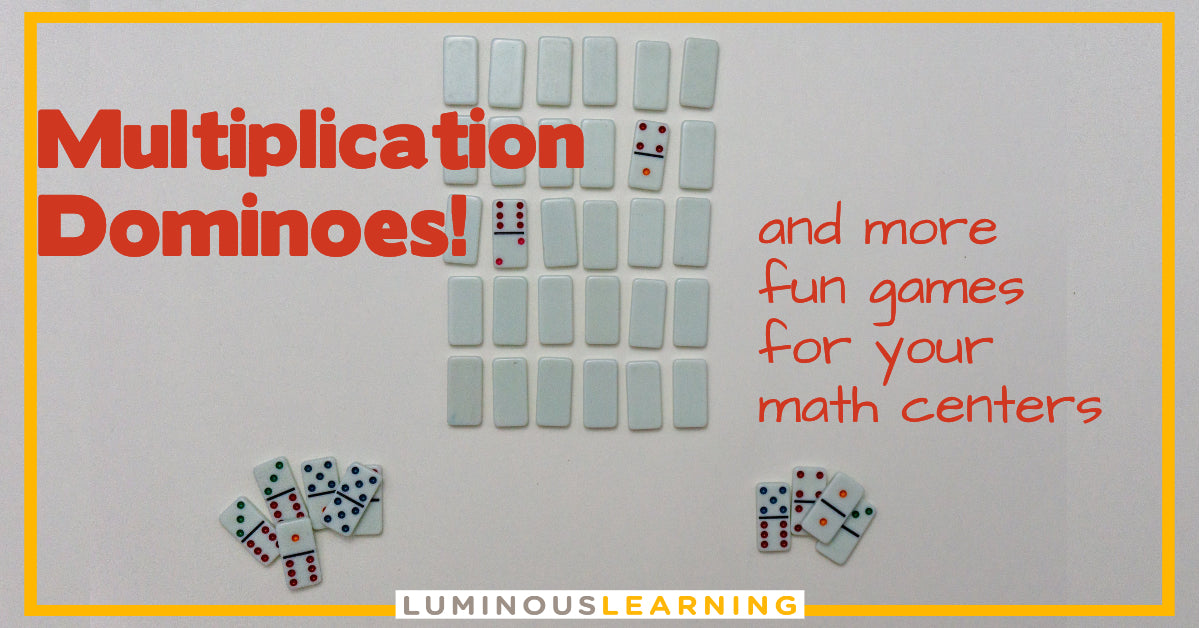Description:

This math games uses dominoes to help students practice their multiplication facts. Students turn over domino tiles and multiply the numbers on either side of the domino piece. Play with 2 - 4 students per group (although if you play with more than 2 students, you may want to combine 2 packs of dominoes.)

Materials:

• set of dominoes

Directions:

• Shuffle the domino tiles and place them face down on the table.
• Each student chooses one domino tile to turn over at the same time.
• The number of either side of the domino tile represents the factors. Students multiply the two numbers to find the product. For example, if I have a 1 and a 6, I multiply 1 x 6 = 6.
• The student with the highest product wins all the domino tiles in this round.
• Go back to step #1. Students continue turning over domino tiles and multiplying the factors. The student who has collected the most number of domino tiles by the end of the game is the winner!

### Jenga multiplication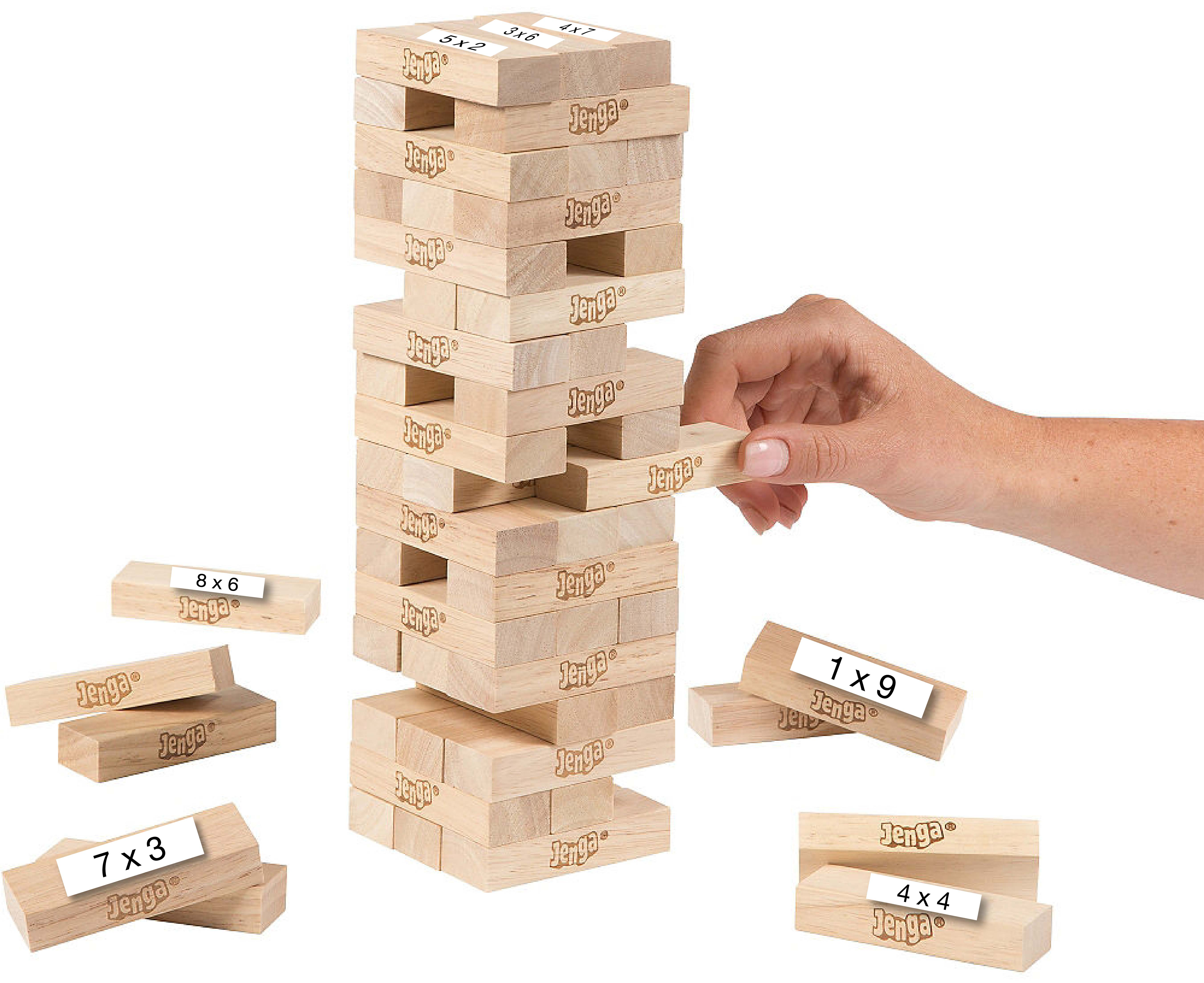Description:

I like to 'mathematize" already existing games in my classroom. You can tape multiplication facts to Jenga tiles and have students solve the problems as they go through the game. Play Jenga Multiplication with 2 - 6 students per group.

Materials:

• Jenga game
• multiplication equations. You can print or hand write multiplication problems on small slips of paper and tape them to the top of each Jenga tile.

Directions:

• The first student chooses a Jenga tile to remove from the tower and place on the top. After the student pulls out the Jenga tile, they must solve the multiplication equation before placing it back on the tower.
• The next student takes a turn and repeats step #1.
• Students continue to solve the multiplication problems taped on the Jenga tiles during their turn.
• Pro tip: You can repurpose Jenga to help your students practice other math facts, as well! Tape addition, subtraction, or division facts onto the tiles to make computation practice more fun.

### Board games multiplication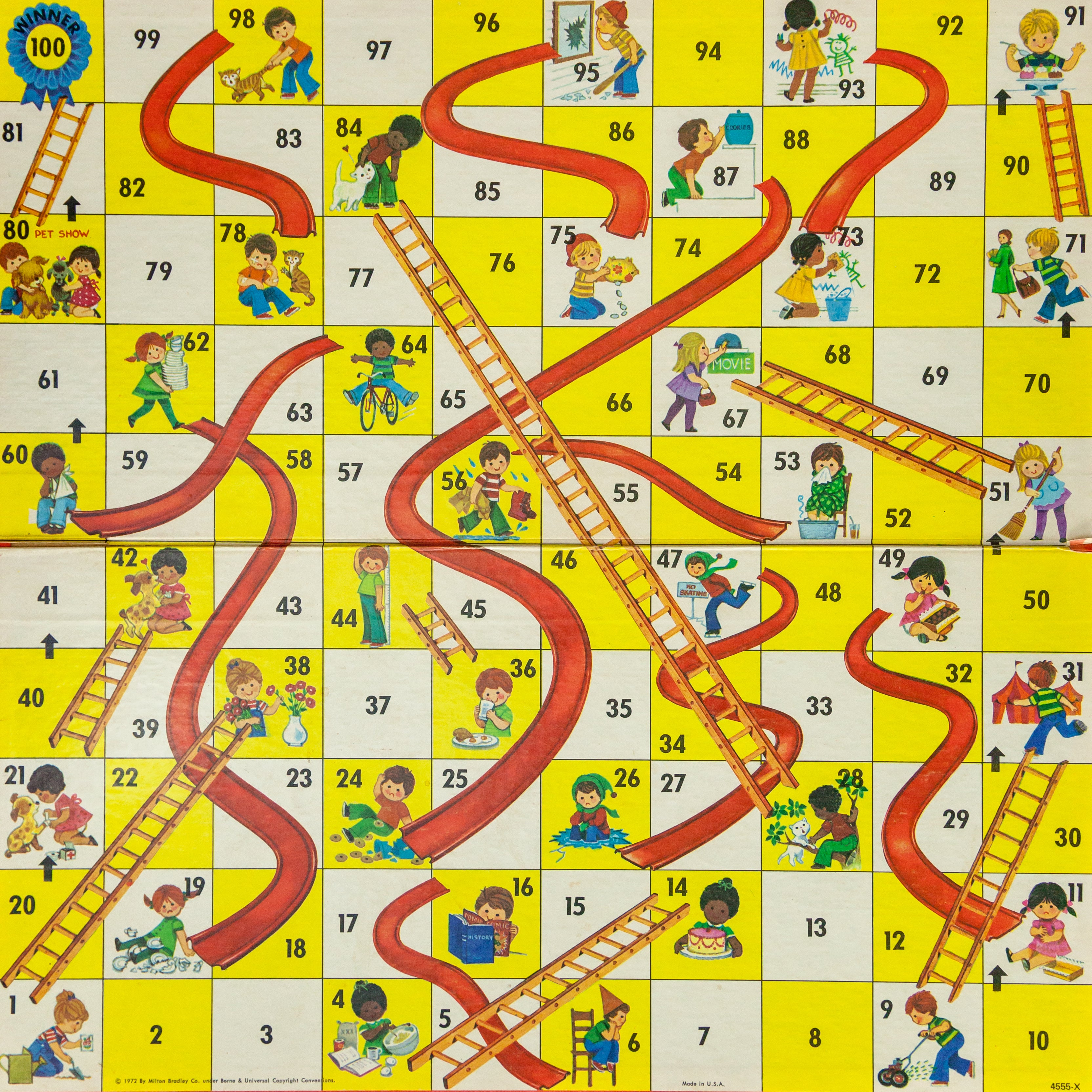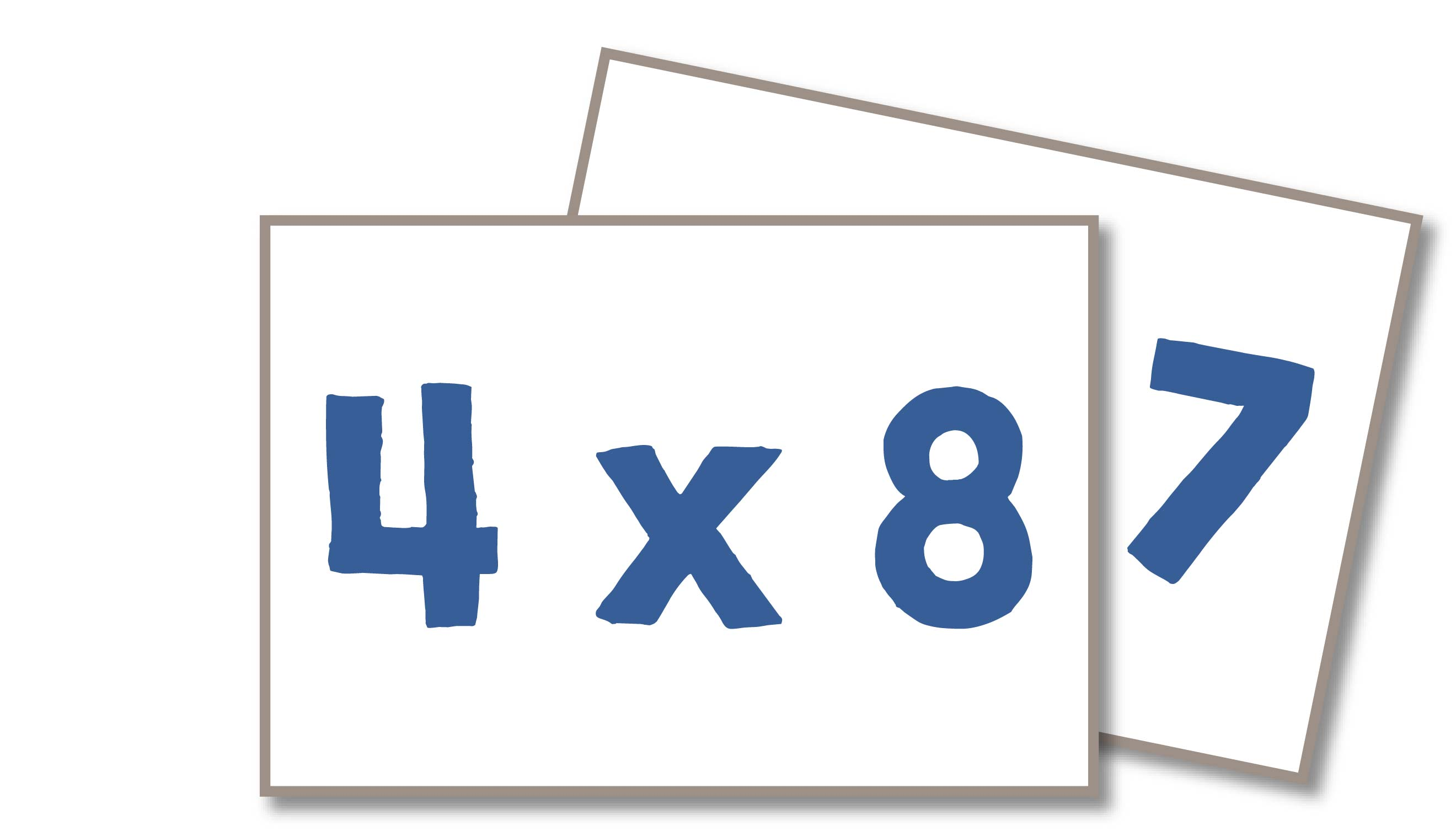Description:

Similar to Jenga, I like to repurpose favorite board games and "mathematize" them for multiplication practice. Chutes and Ladders was always a classroom favorite, even among my older students, although you can use any board game you have in your classroom. Students solve multiplication facts as they progress through the game. Play with 2 - 6 students per group.

Materials:

• multiplication fact cards. You can use flashcards or write multiplication facts on index cards.
• board game. You can use any board game you have in your classroom.

Directions:

• Shuffle multiplication flashcards or index cards and turn them upside down in a pile.
• Each time a student takes a turn, they must first take a multiplication card from the top of the pile and solve the equation. Once they've solved the equation, they can roll the dice and continue playing the game.
• Students take turns turning over a multiplication card and solving the problem at the beginning of their turn.

### WRAPPING IT UP...

I hope you find some of these multiplication games useful for your math centers! These kinds of engaging math games, especially the ones that use manipulatives and drawings, are especially useful for our special education students who need to strengthen their conceptual understanding.

Make sure to download How to Teach Multiplication and Division Ebook below to get a free copy of two multiplication games and one division game.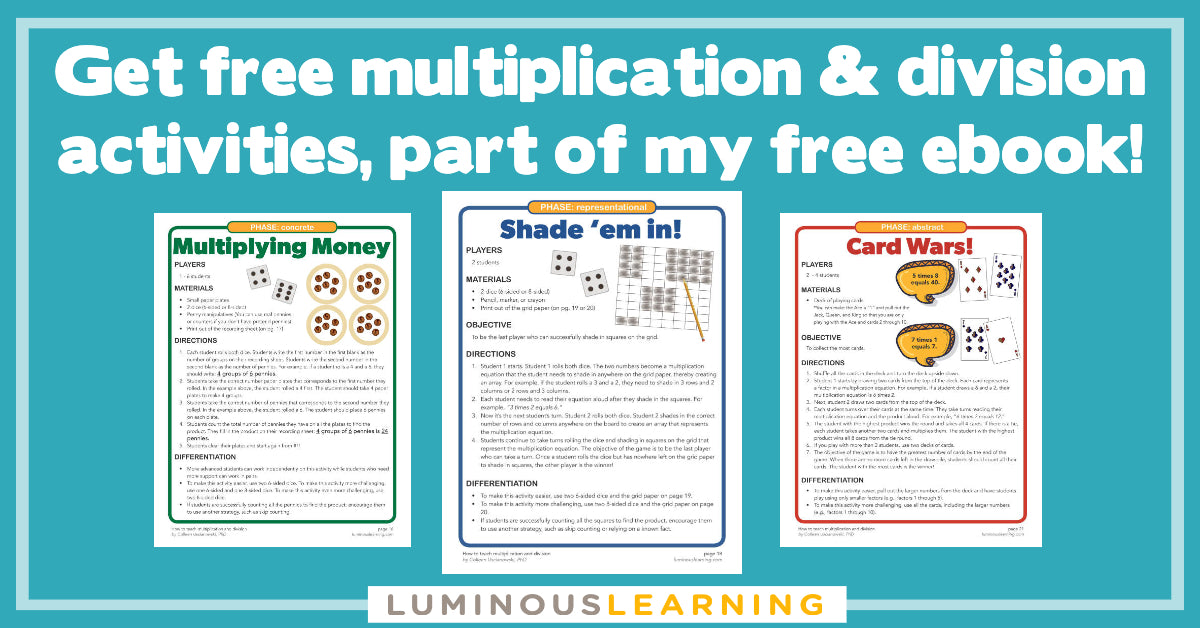# How to Teach Multiplication and Division ebook

Get your FREE 20+ page guide to teaching multiplication in the special education classroom + printable activities!

#### Now, let’s chat in the comments: What are some of your favorite games for teaching multiplication?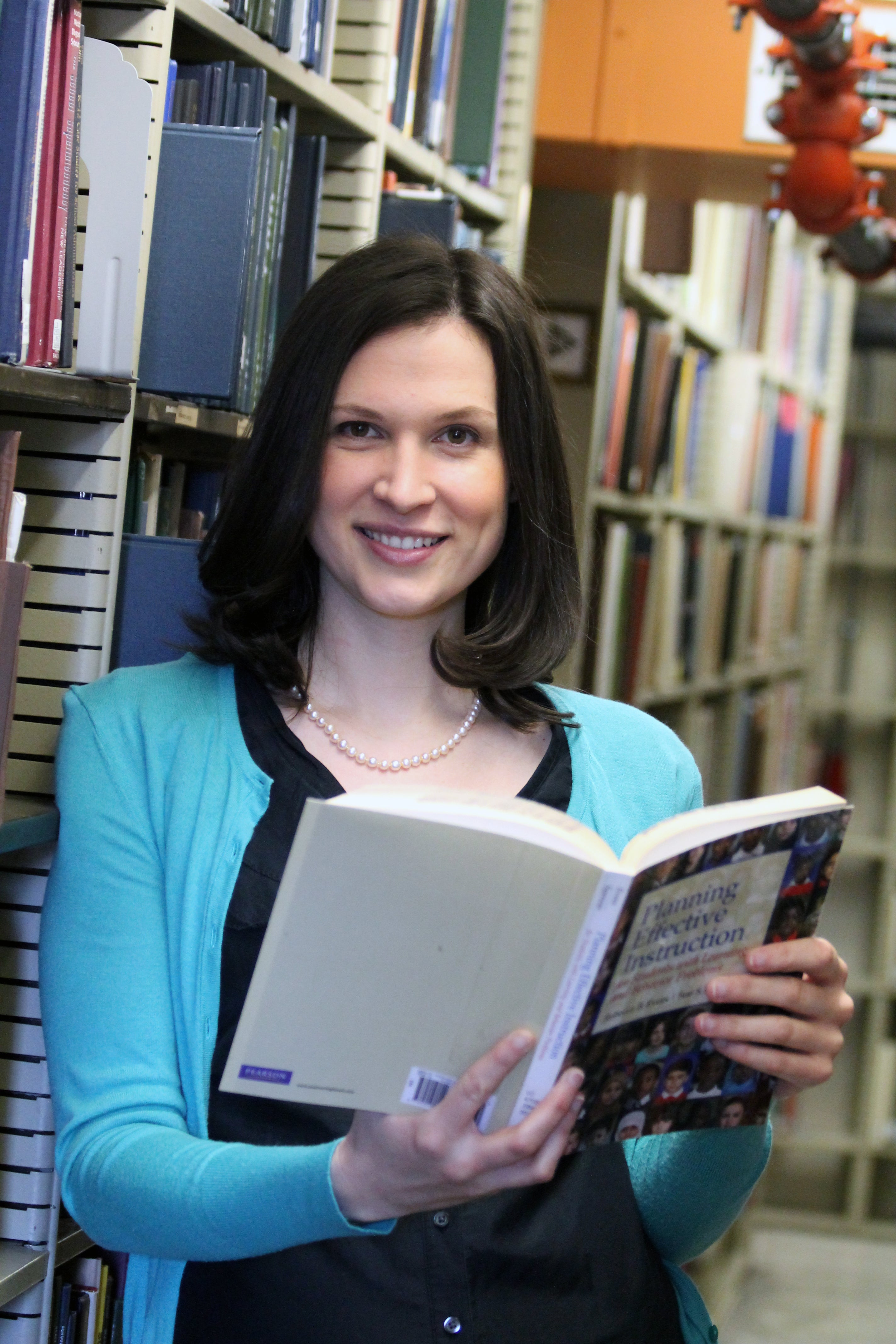### Hi, I'm Colleen

I believe math is for everyone and even our most struggling students can grow their math minds. I help special education teachers improve their math instruction and send their students soaring to new heights!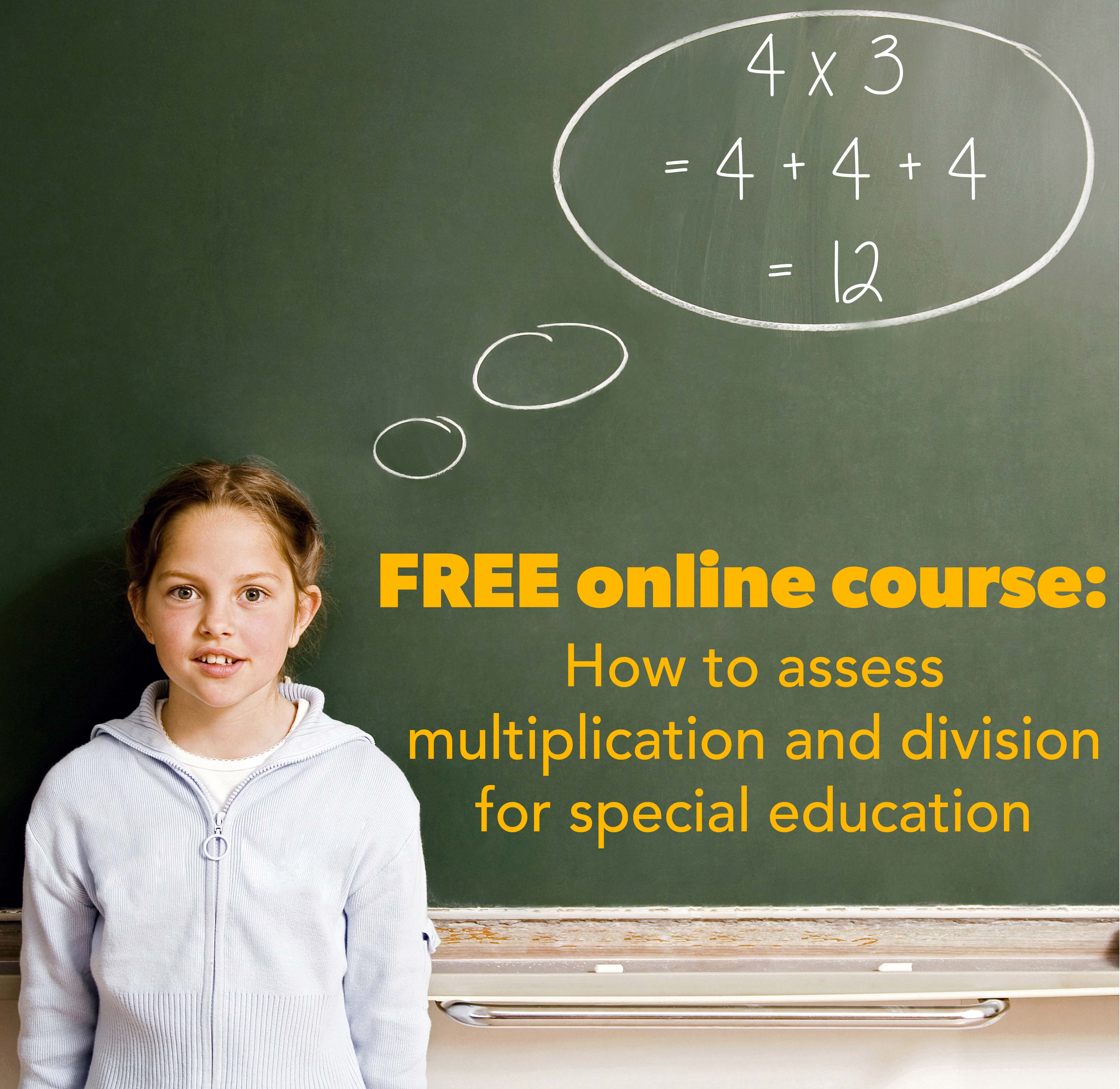## I want to learn about...

### related posts

Your store hasn't published any blog posts yet. A blog can be used to talk about new product launches, tips, or other news you want to share with your customers.

Your store hasn't published any blog posts yet. A blog can be used to talk about new product launches, tips, or other news you want to share with your customers.